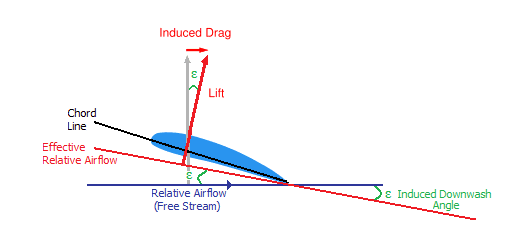# Induced Drag Airfoil: Proportional to Velocity^2?

• GlynnHeeswijk
It lowers the induced drag coefficient, but increases the parasitic drag coefficient. I'm not sure why there is such a focus on separating total drag into induced and parasitic components. In the real world, a wing design is a compromise between lift/(total drag) ratio for a given speed range and cost to manufacture.

#### GlynnHeeswijk

Induced drag of an airfoil should be inversely proportional to velocity^2 wiki states "Since induced drag is inversely proportional to the square of the airspeed" Ok but [Induced Drag = 0.5 * Density * Velocity2 * Wing Area * Induced Drag Coefficient] which is proportional to the veloicty^2 and not inversely proportional and the term CDi which is CDi = (k*CL2)/ (pi*AR) does not have a velocity term thus how can it be inversely proportional?

Last edited:
Induced drag is normally defined as sin(θ) x lift, where θ = effective angle of attack, or θ = effective angle of deflection of air flow relative to the aircraft, which is what the wiki article diagram shows.As airspeed increased, the angle of diversion decreases, so that sin(θ) x lift decreases with speed^2. This is an approximation that starts to deviate from reality once above mach 0.3->0.4, depending on the aircraft.

The wiki article claims that the source of induce drag and downwash (lift) is related to wingtip vortices:

wiki_Source_of_induced_drag.html

However other web sites point out the somewhat obvious fact that induced drag on an 747 at takeoff and landing (slow speed, high amount of induced drag) would rip the wing tips apart if the source of induced drag was solely due to wingtip vortices or wingtip and trailing edge vortices (some airfoils minimize trailing edge vortices for a given load and speed range).

Last edited:
But CDi = (k*CL2)/ (pi*AR) (coefficent of induced drag) doesn't have a velocity term and neither does CL (coefficent of lift) so I don't see why it would change with velocity.

GlynnHeeswijk said:
But CDi = (k*CL2)/ (pi*AR) (coefficent of induced drag) doesn't have a velocity term and neither does CL (coefficent of lift) so I don't see why it would change with velocity.
In level flight, the amount of lift generated is constant, so CL and CDi would decrease with speed^2, a reasonable approximation until you reach speeds above mach 0.3 or 0.4, where a much more complex form of mathematical model is requried.

Jeff Reid said:
In level flight, the amount of lift generated is constant, so CL and CDi would decrease with speed^2, a reasonable approximation until you reach speeds above mach 0.3 or 0.4, where a much more complex form of mathematical model is requried.

That kinda makes sense as you would lower angle of attack to keep the lift constant as velocity increases thus lowering the lift coefficient which would in turn lower the induced drag coefficient.

GlynnHeeswijk said:
That kinda makes sense as you would lower angle of attack to keep the lift constant as velocity increases thus lowering the lift coefficient which would in turn lower the induced drag coefficient.
It lowers the induced drag coefficient, but increases the parasitic drag coefficient. I'm not sure why there is such a focus on separating total drag into induced and parasitic components. In the real world, a wing design is a compromise between lift/(total drag) ratio for a given speed range and cost to manufacture.

## 1. What is induced drag on an airfoil?

Induced drag is a type of drag force that is created when an airfoil generates lift. It is caused by the pressure difference between the upper and lower surfaces of the airfoil, and it acts in the opposite direction of the lift force.

## 2. How is induced drag related to velocity?

Induced drag is directly proportional to the square of the airfoil's velocity. This means that as the velocity increases, the induced drag force also increases, and vice versa.

## 3. What factors affect the amount of induced drag on an airfoil?

The amount of induced drag on an airfoil is affected by several factors, including the angle of attack, the shape and size of the airfoil, and the airfoil's speed. Additionally, the density and viscosity of the air also play a role in determining the amount of induced drag.

## 4. How does an airfoil's shape impact induced drag?

The shape of an airfoil can greatly impact the amount of induced drag it produces. Airfoils with a higher aspect ratio (length/width) tend to produce less induced drag compared to airfoils with a lower aspect ratio. Additionally, airfoils with a curved or elliptical shape tend to have less induced drag than those with a straight or angled shape.

## 5. Can induced drag be reduced or eliminated?

Induced drag is an inevitable consequence of generating lift on an airfoil. However, it can be reduced through various methods such as increasing the aspect ratio, using wingtip devices like winglets, and using a more streamlined airfoil shape. In some cases, induced drag can even be eliminated completely through the use of advanced aerodynamic designs.